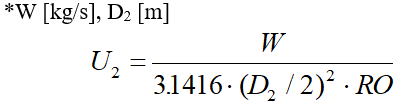Liquid Water - Intergraph Smart Instrumentation - 13.1 - Reference - Hexagon PPM

Integraph Smart Instrumentation Sizing Equations

Language
English (United States)
Product
Intergraph Smart Instrumentation
Search by Category
Reference
Smart Instrumentation Version
13.1
1. Calculate FF: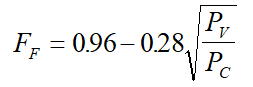2. Calculate n: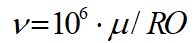3. Calculate Kc :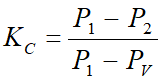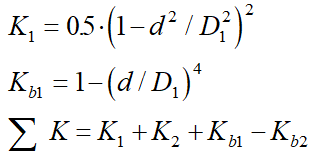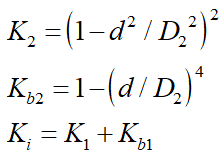5. Calculate first value of CV: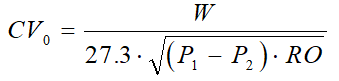6. Calculate Fp: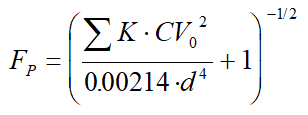7. Calculate Kcv: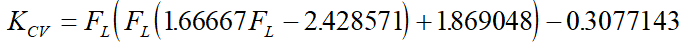8. Calculate FLP: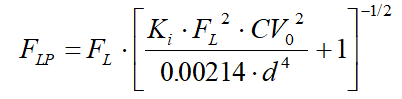9. Calculate Re: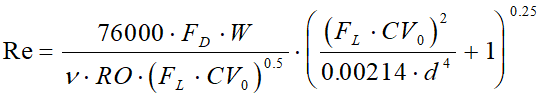10. Calculate DPA: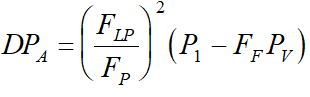11. Calculate Fr:

At this stage, the following cases for different Re are possible:

1. Case Re< - Laminar flow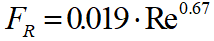2. Case 56 < Re <40000 - Transitional flow

1.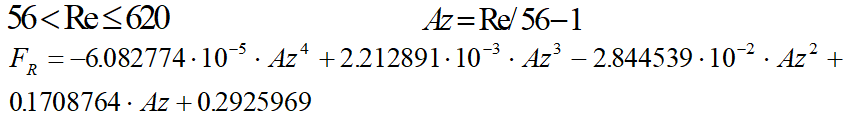2.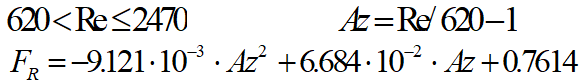3.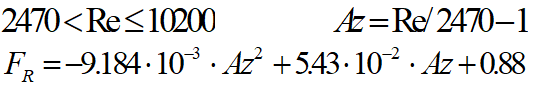4.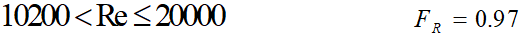5.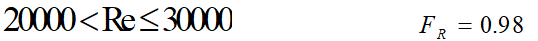6.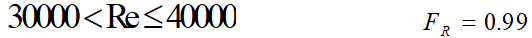3. Case Re>40000 - Turbulent flow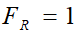12. The condition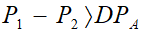determines:

Case A - Cavitation and Flashing

1. Cavitation takes place if P2ñPV, otherwise flashing case takes place.

2. Calculate new CV.

ISA Standard: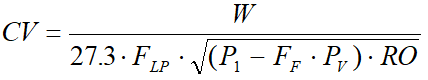IEC Standard: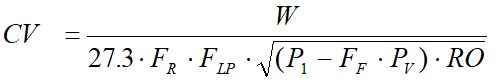Case B (P1 - P2 £ DP A) - Usual

ISA Standard: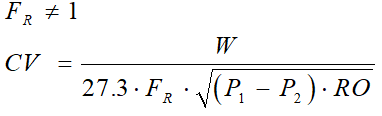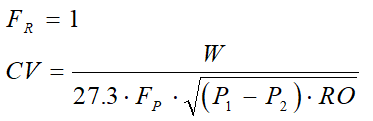IEC Standard: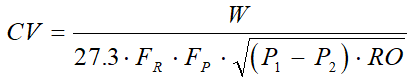13. Calculate the relative change in the CV: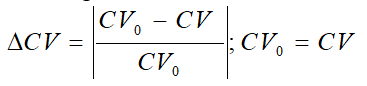14. Steps 5 to 13 are repeated until the relative change in the CV is less than 0.001.

15. Define "Incipient" case. The "Incipient" case takes place if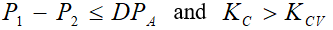16. Calculate outlet pipe velocity: